# [Statistics] Comparison of Three Correlation Coefficients: Pearson, Kendall, Spearman

There are three popular metrics to measure the correlation between two random variables: Pearson's correlation coefficient, Kendall's tau and Spearman's rank correlation coefficient. In this article, I will make a detailed comparison among the three measures and discuss how to choose among them.

# Definition

## Pearson Correlation

Pearson's correlation coefficient is the covariance of the two variables divided by the product of their standard deviations.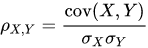The formula forcan be expressed in terms of mean and expectation. Since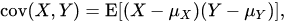the formula forcan also be written as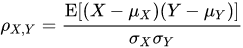## Kendall's Tau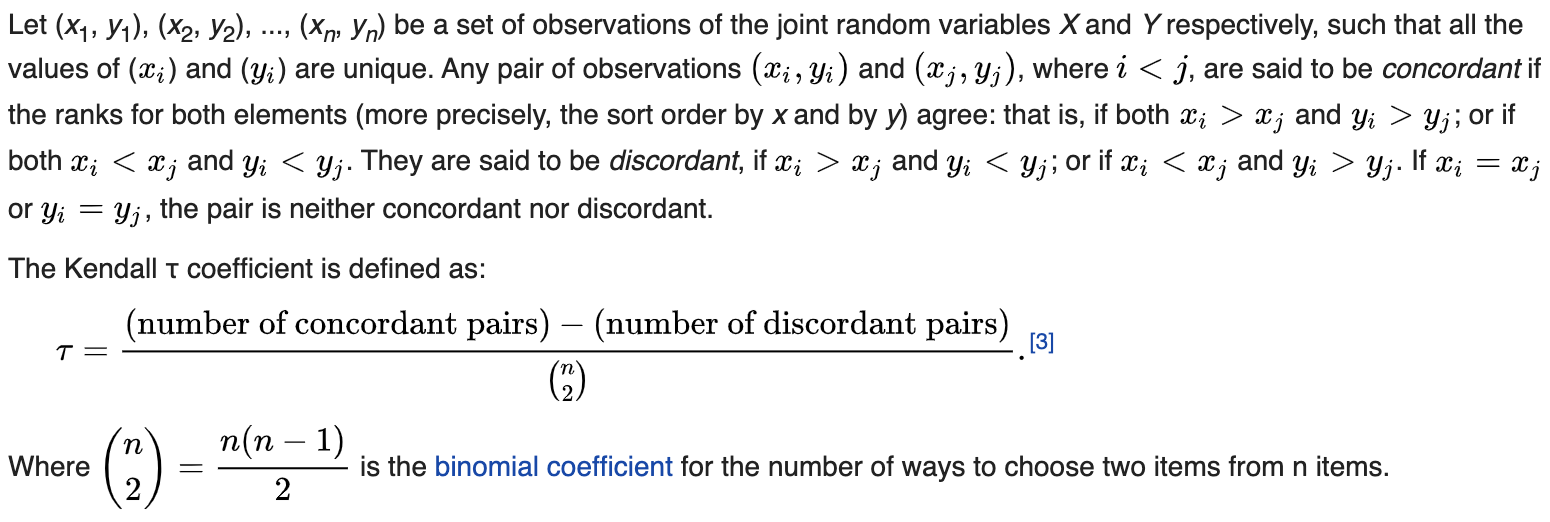An explicit expression for Kendall's rank coefficient is.

## Spearman's Rank Correlation Coefficient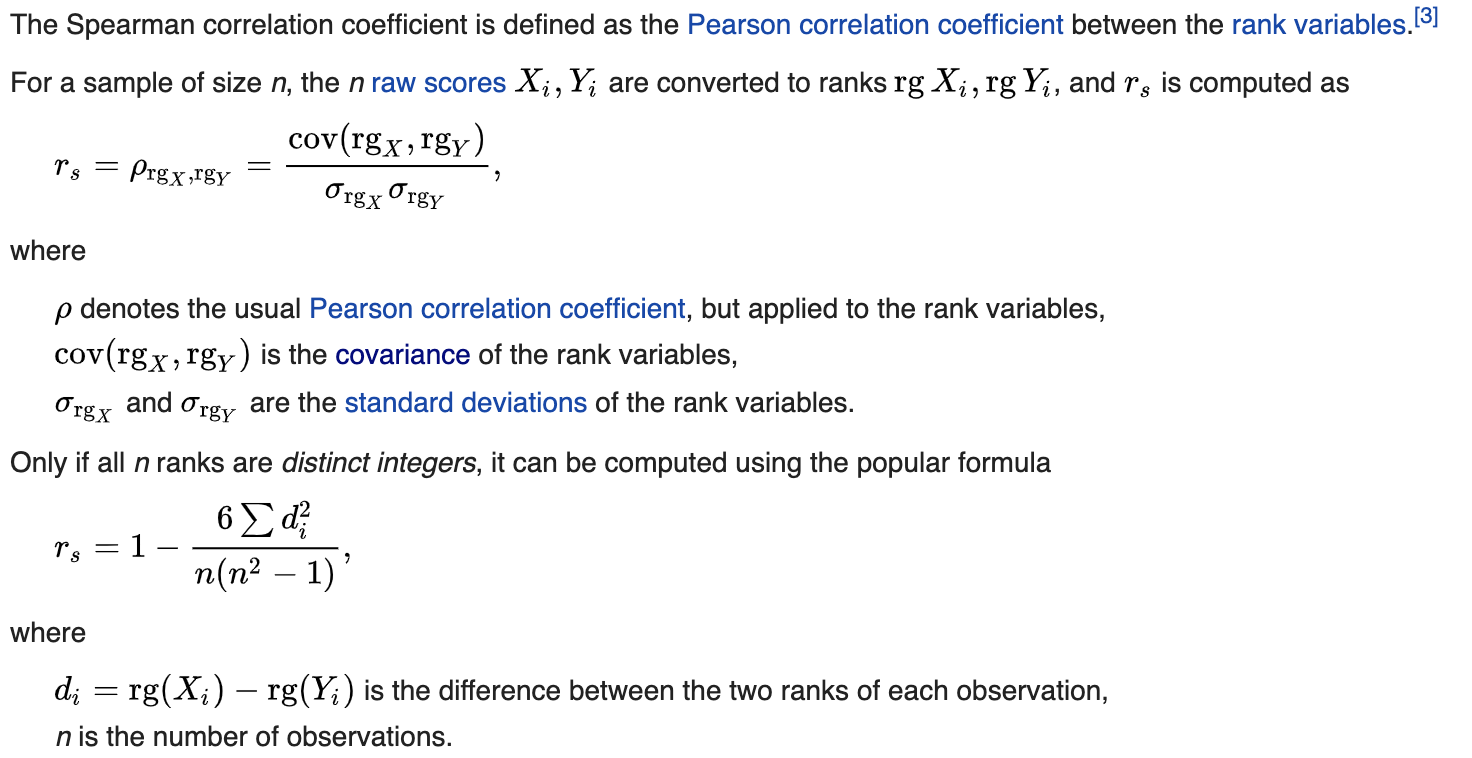# Comparison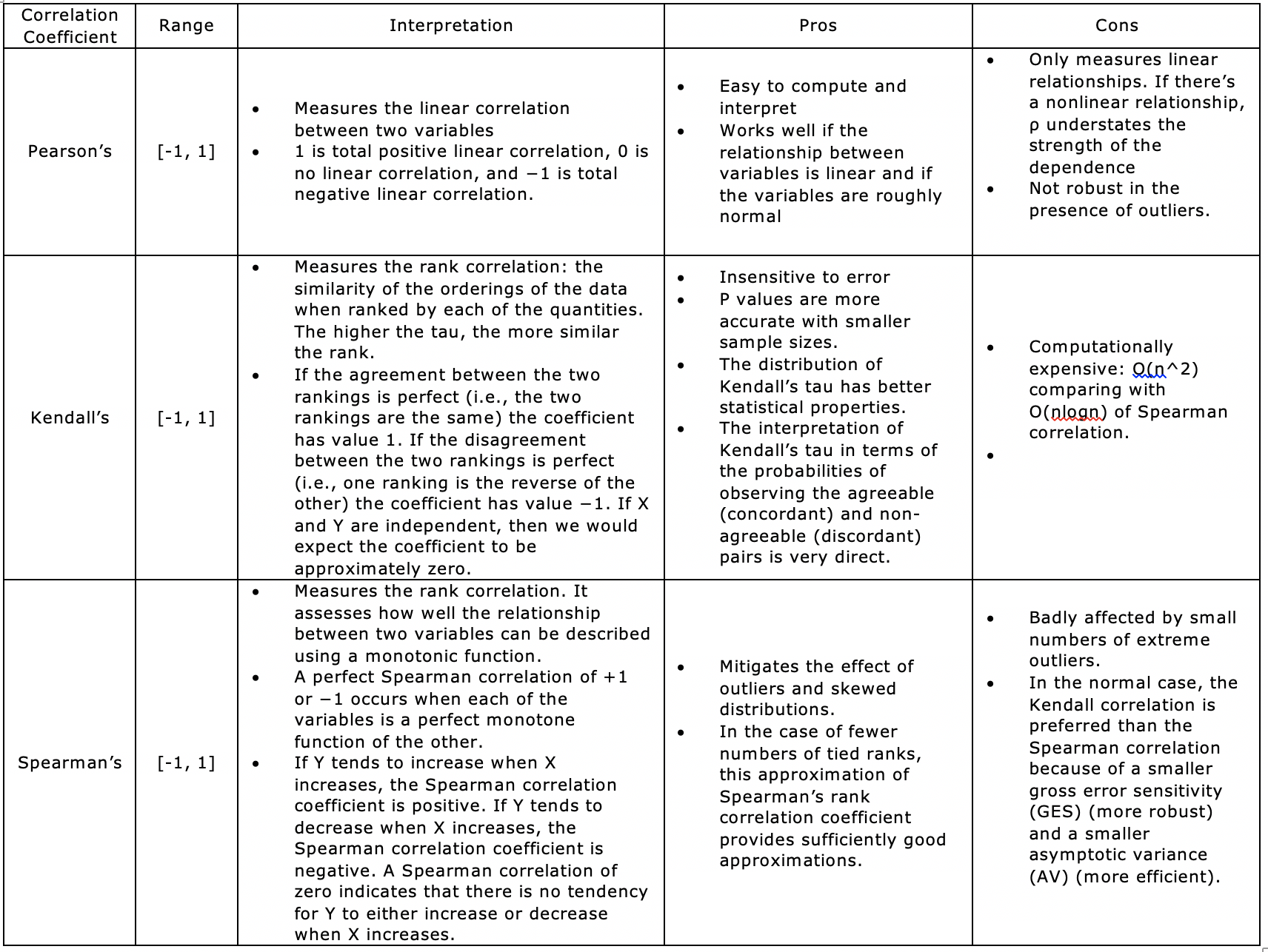# Reference

posted @ 2020-03-04 03:34  Sherrrry  阅读(267)  评论(0编辑  收藏  举报